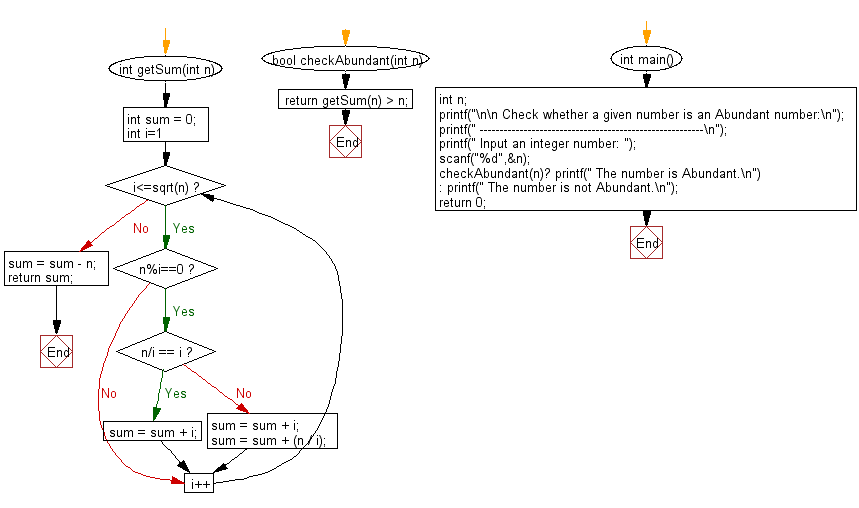﻿ C Program: Check whether a given number is Abundant or not - w3resource# C Exercises: Check whether a given number is Abundant or not

## C Numbers: Exercise-2 with Solution

Write a program in C to check whether a given number is Abundant or not.

Test Data
Input an integer number: 18

Sample Solution:

C Code:

``````# include <stdio.h>
# include <string.h>
# include <stdbool.h>
# include <math.h>

int getSum(int n)
{
int sum = 0;
for (int i=1; i<=sqrt(n); i++)
{
if (n%i==0)
{
if (n/i == i)
sum = sum + i;
else
{
sum = sum + i;
sum = sum + (n / i);
}
}
}
sum = sum - n;
return sum;
}
bool checkAbundant(int n)
{
return getSum(n) > n;

}
int main()
{
int n;
printf("\n\n Check whether a given number is an Abundant number:\n");
printf(" --------------------------------------------------------\n");
printf(" Input an integer number: ");
scanf("%d",&n);

checkAbundant(n)? printf(" The number is Abundant.\n") : printf(" The number is not Abundant.\n");
return 0;
}
```
```

Sample Output:

``` Input an integer number: 18
The number is Abundant.
```

Pictorial Presentation:Flowchart:C Programming Code Editor:

What is the difficulty level of this exercise?

Test your Programming skills with w3resource's quiz.

﻿

## C Programming: Tips of the Day

Why do C and C++ compilers allow array lengths in function signatures when they're never enforced?

It is a quirk of the syntax for passing arrays to functions.

Actually it is not possible to pass an array in C. If you write syntax that looks like it should pass the array, what actually happens is that a pointer to the first element of the array is passed instead.

Since the pointer does not include any length information, the contents of your [] in the function formal parameter list are actually ignored.

Ref : https://bit.ly/3fhlvdH